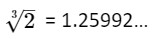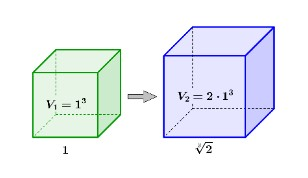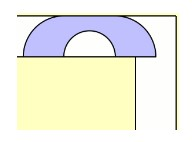# Five Lesser Known Mathematical Constants

Pi is just one of many interesting mathematical constants which you can introduce your pupils to. Here are five more unusual ones which you might like to bring into your classroom to get your students thinking.

### Delian Constant

Next time you get an answer of the cube root of two on your calculator, you could call it by its proper title – The Delian Constant.
Delian Constant =According to legend, the people of Delos were told by an Oracle to double the volume of their cube shaped altar in order to defeat a plague. They asked Plato to help, who realised that this involved calculating the cube root of two.  It took until 1837 for someone to show that the cube root of two is not constructible using a straight edge and compass. Those Delians never stood a chance in doubling the volume of their altar.Image credit: Doubling the cube

### Sofa ConstantImage Credit: Photo by Hal Gatewood on Unsplash

If you have ever struggled to move furniture, then the sofa constant is for you. It specifies the biggest possible sofa area that can be moved around a corner, in a corridor of width 1. (Assuming you can’t tip the sofa up on end.) Unfortunately, nobody has yet found the exact value of the Sofa Constant - this is currently what is called an open mathematical problem. Mathematicians have however found lower and upper bounds, which show it to be somewhere between 1.57 and 2.37.  This particular attempt at a solution doesn’t look very comfortable to sit on…Image Credit: Moving sofa problem

### Kaprekar’s ConstantImage Credit: D. R. Kaprekar
Dattatreya Ramchandra Kaprekar (1905 – 1986) – Indian Mathematician who discovered the Kaprekar constant in 1949.
Think of a four-digit number (any whole number is fine, as long as at least two of the digits are different).
For example, 9114
Put the digits in order, highest to lowest.
9411
Then put the digits in order, lowest to highest.
1149
Then subtract these two numbers.
9411 – 1149 = 8262
Then repeat the process:
2nd Iteration: 8622 – 2268 = 6354
Repeat again:
3rd Iteration: 6543 – 3456 = 3087
4th Iteration: 8730 – 0378 = 8352
5th Iteration: 8532 – 2358 = 6174